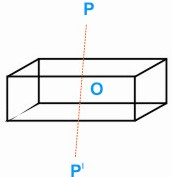HOME MATH DICTIONARY DOWNLOAD FEEDBACK DISCLAIMER
 Question: What is Point Symmetry ? Answer: Consider a cuboid with a small cube cut out of a pair of opposite corners. This modified block has no axis of rotational symmetry or plane of symmetry, but it has symmetry about its center O. For every point P on the block there is another point P' such that O is the mid-point of PP'. This kind of symmetry is called point symmetry. A two-dimensional object can only have point symmetry when it has rotational symmetry about its center of even order. Example of a Point Symmetry: